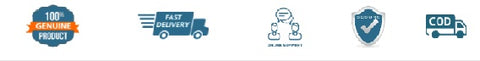# NCERT Exemplar Problems Mathematics for Class 12 - latest edition as per NCERT/CBSE

SKU:3MNCERT316
Price : Rs. 159.00
Sale price Rs. 159.00 Price : Rs. 177.00
Sold out
Unit price
per
Shipping calculated at checkout.

Mathematics
Unit 1(Relations And Functions)
Unit 2(Inverse Trigonometric Functions)
Unit 3(Matrices)
Unit 4(Determinants)
Unit 5(Continuity And Differentiability)
Unit 6(Application Of Derivatives)
Unit 7(Integrals)
Unit 8(Application Of Integrals)
Unit 9(Differential Equations)
Unit 10(Vector Algebra)
Unit 11(Three Dimensional Geometry)
Unit 12(Linear Programming)
Unit 13(Probability)
(Design Of The Question Paper-Set I)
(Design Of The Question Paper-Set II)

(All books are 100℅ original and as per the latest NCERT syllabus. Prices includes Rs. 32 per book mandatory binding charges.)